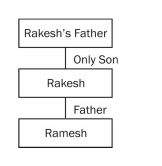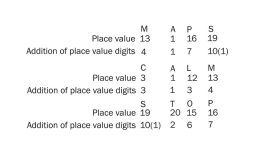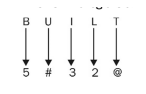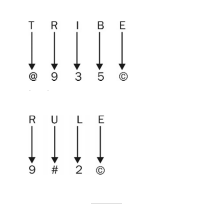# RRB ALP 2018 Practice Test Papers | Reasoning Questions (Day-13)

Dear Aspirants, Here we have given the Important RRB ALP & Technicians Exam 2018 Practice Test Papers. Candidates those who are preparing for RRB ALP 2018 can practice these Reasoning Questions to get more confidence to Crack RRB 2018 Examination.

[WpProQuiz 1611]

Click “Start Quiz” to attend these Questions and view Solutions

1).  Choose the odd one out.

1. Assam
2. Karnataka
3. Manipur
4. Sikkim

2).  Choose the odd one out.

1. 28
2. 50
3. 49
4. 63

3). The father of Ramesh is the only son of Rakesh’s father. How is Ramesh related to Rakesh?

1. Father
2. Son
3. Uncle
4. Grandfather

4). 11, 13, 16, 20,?

1. 25
2. 26
3. 28
4. 27

5). Select set of letters from the given alternatives which when sequentially placed at the gaps in the given letter series, complete it. xx_zzyx_yzzyxxy_zy_xyzz_

1. yxzxy
2. yxzyx
3. yxxyz
4. xxzyy

6). In a certain code ‘MAPS’ is written as 4171 and ‘CALM’ is written as 3134. How would ‘STOP’ be written in the same code?

1. 2167
2. 2176
3. 1276
4. 1267

7). Choose the pair which is different from the rest.

1. Tree: Stem
2. Face: Eye
3. Chair: Table
4. Plant: Flower

8). If ‘−’ stands for ‘×’, ‘×’ stands for ‘+’, ‘+’ stands for ‘÷’, and ‘÷’ stands for ‘−’, then 42 + 7 – 6 ÷ 5 × 14 =?

1. 36
2. 40
3. 43
4. 45

9). In a certain code, ‘BUILT’ is written as ‘5#[email protected]’ and ‘TRIBE’ is written as ‘@935©’. In the same code, ‘RULE’ will be written as

1. [email protected]#
4. [email protected]

10). Game: Field:: Cinema:?

(A) Hall

(B) Stage

(C) Screen

(D) Drama

Explanation:

Except Karnataka, all others are north eastern states in India.

All others are divisible by 7, except 50.Only son of Rakesh’s father is Rakesh only. Therefore, father of Ramesh is Rakesh.

The terms of the given series follow the pattern as: 11 + 2 = 13 13 + 3 = 16 16 + 4 = 20 So, the required number = 20 + 5 = 25

The given series is formed by repeating ‘xxyzzy’.Except the pair ‘Chair: Table’, each second word in the given pairs is a part of the first word.

By putting the correct symbols, the equation “42 + 7 – 6 ÷ 5 × 14” would be written as “42 ÷ 7 × 6 – 5 + 14” and calculated as: = 42 ÷ 7 × 6 – 5 + 14 = 6 × 6 – 5 + 14 = 36 – 5 + 14 = 45As the place of game is field similarly the place of cinema is ‘Hall’.

RRB ALP 2018 Practice Test Papers | Reasoning Questions (Day-1)

RRB ALP 2018 Practice Test Papers | Reasoning Questions (Day-2)

RRB ALP 2018 Practice Test Papers | Reasoning Questions (Day-3)

RRB ALP 2018 Practice Test Papers | Reasoning Questions (Day-4)

RRB ALP 2018 Practice Test Papers | Reasoning Questions (Day-5)

RRB ALP 2018 Practice Test Papers | Reasoning Questions (Day-6)

RRB ALP 2018 Practice Test Papers | Reasoning Questions (Day-7)

RRB ALP 2018 Practice Test Papers | Reasoning Questions (Day-8)

RRB ALP 2018 Practice Test Papers | Reasoning Questions (Day-9)

RRB ALP 2018 Practice Test Papers | Reasoning Questions (Day-10)

RRB ALP 2018 Practice Test Papers | Reasoning Questions (Day-11)

RRB ALP 2018 Practice Test Papers | Reasoning Questions (Day-12)